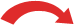# Greatest Common Factor

The highest number that divides exactly into two or more numbers.
It is the "greatest" thing for simplifying fractions!### Greatest Common Factor of 12 and 16

1. Find all the Factors of each number,
2. Circle the Common factors,
3. Choose the Greatest of those

## So ... what is a "Factor" ?

Factors are numbers we can multiply together to get another number:A number can have many factors:

Factors of 12 are 1, 2, 3, 4, 6 and 12 ...

... because 2 × 6 = 12, or 4 × 3 = 12, or 1 × 12 = 12.

(Read how to find All the Factors of a Number. In our case we don't need the negative ones.)

## What is a "Common Factor" ?

Say we have worked out the factors of two numbers:

### Example: Factors of 12 and 30

 Factors of 12 are 1, 2, 3, 4, 6 and 12 Factors of 30 are 1, 2, 3, 5, 6, 10, 15 and 30

Then the common factors are those that are found in both lists:

• Notice that 1, 2, 3 and 6 appear in both lists?
• So, the common factors of 12 and 30 are: 1, 2, 3 and 6

It is a common factor when it is a factor of two (or more) numbers.

Here is another example with three numbers:

### Example: The common factors of 15, 30 and 105

 Factors of 15 are 1, 3, 5, and 15 Factors of 30 are 1, 2, 3, 5, 6, 10, 15 and 30 Factors of 105 are 1, 3, 5, 7, 15, 21, 35 and 105

The factors that are common to all three numbers are 1, 3, 5 and 15

In other words, the common factors of 15, 30 and 105 are 1, 3, 5 and 15

## What is the "Greatest Common Factor" ?

It is simply the largest of the common factors.

In our previous example, the largest of the common factors is 15, so the Greatest Common Factor of 15, 30 and 105 is 15

The "Greatest Common Factor" is the largest of the common factors (of two or more numbers)

## Why is this Useful?

One of the most useful things is when we want to simplify a fraction:

### Example: How can we simplify 1230 ?

Earlier we found that the Common Factors of 12 and 30 are 1, 2, 3 and 6, and so the Greatest Common Factor is 6.

So the largest number we can divide both 12 and 30 exactly by is 6, like this:

 ÷ 61230 = 25÷ 6

The Greatest Common Factor of 12 and 30 is 6.

And so 1230 can be simplified to 25

## Finding the Greatest Common Factor

Here are three ways:

1. We can:

• find all factors of both numbers (use the All Factors Calculator),
• then find the ones that are common to both, and
• then choose the greatest.

Example:

Two Numbers Factors Common Factors Greatest
Common Factor
Example Simplified
Fraction
9 and 12  9: 1, 3, 9
12: 1, 2, 3, 4, 6, 12
1, 3 3 912 = 34

And another example:

Two Numbers Factors Common Factors Greatest
Common Factor
Example Simplified
Fraction
6 and 18  6: 1, 2, 3, 6
18: 1, 2, 3, 6, 9, 18
1, 2, 3, 6 6 618 = 13

2. Or we can find the prime factors and combine the common ones together:

Two Numbers Thinking ... Greatest
Common Factor
Example Simplified
Fraction
24 and 108 2 × 2 × 2 × 3 = 24, and
2 × 2 × 3 × 3 × 3 = 108
2 × 2 × 3 = 12 24108 = 29

3. Or sometimes we can just play around with the factors until we discover it:

Two Numbers Thinking ... Greatest
Common Factor
Example Simplified
Fraction
9 and 12 3 × 3 = 9 and 3 × 4 = 12 3 912 = 34

But in that case we must check that we have found the greatest common factor.

## Greatest Common Factor Calculator

OK, there is also a really easy method: we can use the Greatest Common Factor Calculator to find it automatically.

## Other Names

The "Greatest Common Factor" is often abbreviated to "GCF", and is also known as:

• the "Greatest Common Divisor (GCD)", or
• the "Highest Common Factor (HCF)"